## Dynamical System

A means of describing how one state develops into another state over the course of time. Technically, a dynamical system is a smooth action of the reals or the Integers on another object (usually a Manifold). When the reals are acting, the system is called a continuous dynamical system, and when the Integers are acting, the system is called a discrete dynamical system. If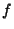is any Continuous Function, then the evolution of a variablecan be given by the formula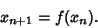(1)

This equation can also be viewed as a difference equation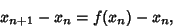(2)

so defining(3)

gives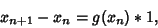(4)

which can be read aschanges by 1 unit,changes by.'' This is the discrete analog of the Differential Equation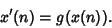(5)

See also Anosov Diffeomorphism, Anosov Flow, Axiom A Diffeomorphism, Axiom A Flow, Bifurcation Theory, Chaos, Ergodic Theory, Geodesic Flow

References

Aoki, N. and Hiraide, K. Topological Theory of Dynamical Systems. Amsterdam, Netherlands: North-Holland, 1994.

Golubitsky, M. Introduction to Applied Nonlinear Dynamical Systems and Chaos. New York: Springer-Verlag, 1997.

Guckenheimer, J. and Holmes, P. Nonlinear Oscillations, Dynamical Systems, and Bifurcations of Vector Fields, 3rd ed. New York: Springer-Verlag, 1997.

Lichtenberg, A. and Lieberman, M. Regular and Stochastic Motion, 2nd ed. New York: Springer-Verlag, 1994.

Ott, E. Chaos in Dynamical Systems. New York: Cambridge University Press, 1993.

Rasband, S. N. Chaotic Dynamics of Nonlinear Systems. New York: Wiley, 1990.

Strogatz, S. H. Nonlinear Dynamics and Chaos, with Applications to Physics, Biology, Chemistry, and Engineering. Reading, MA: Addison-Wesley, 1994.

Tabor, M. Chaos and Integrability in Nonlinear Dynamics: An Introduction. New York: Wiley, 1989.